×#### Thank you for registering.

One of our academic counsellors will contact you within 1 working day.

Click to Chat

1800-1023-196

+91-120-4616500

CART 0

• 0

MY CART (5)

Use Coupon: CART20 and get 20% off on all online Study Material

ITEM
DETAILS
MRP
DISCOUNT
FINAL PRICE
Total Price: Rs.

There are no items in this cart.
Continue Shopping• Complete JEE Main/Advanced Course and Test Series
• OFFERED PRICE: Rs. 15,900
• View Details

```Chapter 4: Triangles Exercise – 4.5

Question: 1

In fig. given below ΔACB ∼ ΔAPQ. If BC = 8 cm, PQ = 4 cm, BA = 6.5 cm, and AP = 2.8 cm find CA and AQ.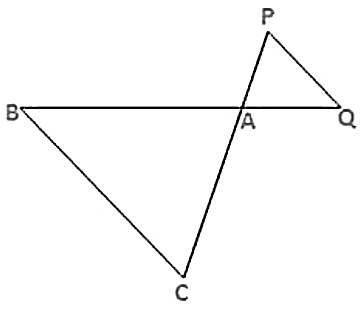Solution:

Given,

ΔACB ∼ ΔAPQ

BC = 8 cm, PQ = 4 cm, BA = 6.5 cm, and AP = 2.8 cm

We need to find CA and AQ

Since, ΔACB ∼ ΔAPQAQ = 3.25 cm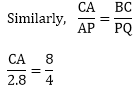CA = 2.8 × 2

CA = 5.6 cm

Therefore, CA = 5.6 cm and AQ = 3.25 cm.

Question: 2

In fig. given, AB ∥ QR, find the length of PB.Solution:

Given,

AB ∥ PB

AB = 3 cm, QR = 9 cm and PR = 6 cm

We need to find out PB,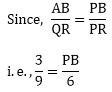PB = 2 cm

Question: 3

In fig. given, XY∥ BC. Find the length of XY.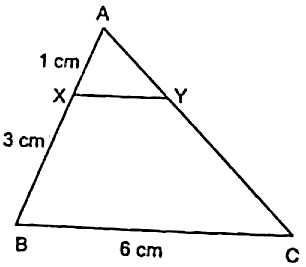Solution:

Given,

XY ∥ BC

AX = 1 cm, XB = 3 cm, and BC = 6 cm

We need to find XY,

Since, ΔAXY ∼ ΔABCXY = 1.5 cm

Question: 4

In a right-angled triangle with sides a and b and hypotenuse c, the altitude drawn on the hypotenuse is x. Prove that ab = cx.

Solution:Let the ΔABC be a right angle triangle having sides a and b and hypotenuse c. BD is the altitude drawn on the hypotenuse AC

We need to prove ab = cx

Since, the altitude is perpendicular on the hypotenuse, both the triangles are similar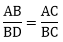a/x = c/b

xc = ab

∴ ab = cx

Question: 5

In fig. given, ∠ABC = 90 and BD⊥AC. If BD = 8 cm, and AD = 4 cm, find CD.Solution:

Given,

∠ABC = 90 and BD⊥AC
When, BD = 8 cm, AD = 4 cm, we need to find CD.

Since, ABC is a right angled triangle and BD⊥AC.

So, ΔDBA ∼ ΔDCB (A-A similarity)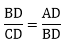(8)2 = 4 × DC

DC = 64/4 = 16 cm

∴ CD = 16 cm

Question: 6

In fig. given, ∠ABC = 90 and BD ⊥ AC. If AC = 5.7 cm, BD = 3.8 cm and CD = 5.4 cm, Find BC.Solution:

Given:  BD ⊥ AC. AC = 5.7 cm, BD = 3.8 cm and CD = 5.4 cm, and ∠ABC = 90.

We need to find BC,

Since, ΔABC ∼ ΔBDC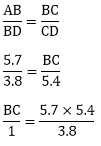BC = 8.1 cm

Question: 7

In the fig. given, DE ∥ BC such that AE = (1/4) AC. If AB = 6 cm, find AD.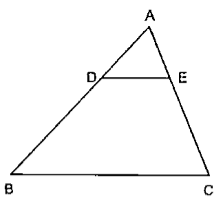Solution:

Given, DE ∥ BC and AE = (1/4) AC and AB = 6 cm.Question: 8

In the fig. given, if AB ⊥ BC, DC ⊥ BC, and DE ⊥ AC, prove that ΔCED ∼ ΔABCSolution:

Given, AB ⊥ BC, DC ⊥ BC, and DE ⊥ AC

We need to prove that ΔCED∼ ΔABC

Now,

In ΔABC and ΔCED

∠B = ∠E = 90   (given)

∠A = ∠ECD  (alternate angles)

So, ΔCED∼ΔABC (A-A similarity)

Question: 9Diagonals AC and BD of a trapezium ABCD with AB∥DC intersect each other at the point O. Using similarity criterion for two triangles, show that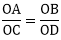Solution:

Given trapezium ABCD with AB∥DC. OC is the point of intersection of AC and BD.

We need to proveNow, in ΔAOB and ΔCOD

∠AOB  = ∠COD    (VOA)

∠OAB   = ∠OCD   (alternate angles)

Therefore, ΔAOB ∼ ΔCOD

Therefore,(corresponding sides are proportional)

Question: 10

If Δ ABC and Δ AMP are two right angled triangles, at angle B and M, respectively. Such that ∠MAP = ∠BAC. Prove that:

(i) ΔABC∼ΔAMPSolution: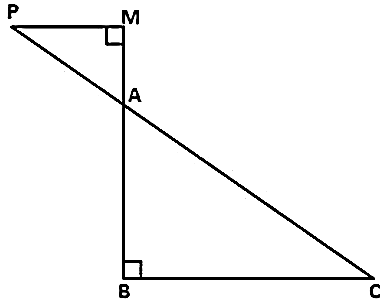(i)  Given Δ ABC and Δ AMP are the two right angled triangle.

∠MAP = ∠BAC   (given)

∠AMP∠AMP = ∠B = 90

ΔABC ∼ ΔAMP    (A-A similarity)

(ii) ΔABC – ΔAMP

So,(corresponding sides are proportional)

Question: 11

A vertical stick 10 cm long casts a shadow 8 cm long. At the same time, a tower casts a shadow 30 m long. Determine the height of the tower.

Solution: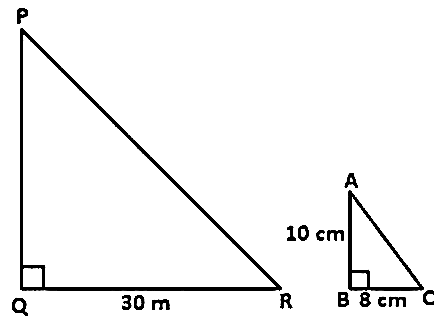We need to find the height of PQ.

Now, ΔABC ∼ ΔPQR  (A – A similarity)PQ = 37.5 m

Question: 12

In fig. given, ∠A  = ∠CED, prove that ΔCAB ∼ ΔCED. Also find the value of x.Solution:

Comparing Δ CAB and ΔCED ΔCAB and ΔCED(Similar triangles have corresponding sides in the same proportions)x = 6 cm

Question: 13

The perimeters of two similar triangles are 25 cm and 15 cm, respect. If one side of the first triangle is 9 cm, what is the corresponding side of the other triangle?

Solution:

Given perimeter of two similar triangles are 25 cm, 15 cm and one side 9 cm

We need to find the other side.

Let the corresponding side of other triangle be x cm

Since ratio of perimeter = ratio of corresponding side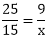25 × x = 9 × 15

x = 135/25

x = 5.4 cm

Question: 14

In ΔABC and ΔDEF, it is being given that AB = 5 cm, BC = 4 cm, CA = 4.2 cm, DE = 10 cm, EF = 8 cm, and FD = 8.4 cm. If AL ⊥ BC, DM ⊥ EF, find AL: Dm.

Solution:

Given AB = 5 cm, BC = 4 cm, CA = 4.2 cm, DE = 10 cm, EF = 8 cm, and FD = 8.4 cm

We need to find AL: DM

Since, both triangles are similar,Here, we use the result that in similar triangle the ratio of corresponding altitude is same as the ratio of the corresponding sides.

Therefore,   AL: DM = 1: 2

Question: 15

D and E are the points on the sides AB and AC respectively, of a ΔABC such that AD = 8 cm, DB = 12 cm, AE = 6 cm, and CE = 9 cm. Prove that BC = 5/2 DE.

Solution:Given AD = 8 cm, AE = 6 cm, and CE = 9 cm

We need to prove that,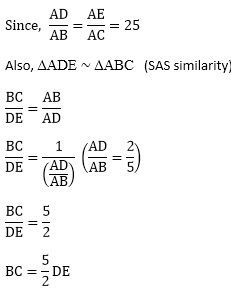Question: 16

D is the midpoint of side BC of a ΔABC. AD is bisected at the point E and BE produced cuts AC at the point X. Prove that BE: EX = 3 : 1

Solution:

ABC is a triangle in which D is the midpoint of BC, E is the midpoint of AD. BE produced meets AC at X.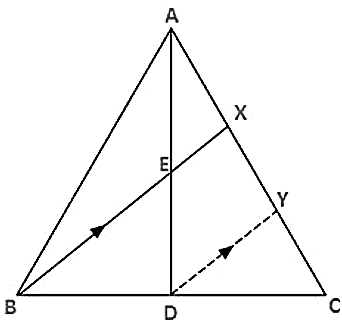We need to prove BE: EX = 3:1

In Δ BCX  and  ΔDCY

∠CBX  = ΔCBY   (corresponding angles)

∠CXB  = ΔCYD   (corresponding angles)

ΔBCX ∼ ΔDCY   (angle-angle similarity)

We know that corresponding sides of similar sides of similar triangles are proportional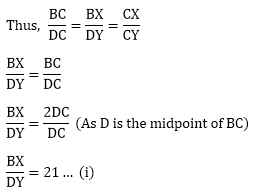∠AXE = ΔAYD  (corresponding angles)

We know that corresponding sides of similar sides of similar triangles are proportionalDividing eqn. (i) by eqn. (ii)BX = 4EX

BE + EX = 4EX

BE = 3EX

BE: EX = 3:1

Question: 17

ABCD is a parallelogram and APQ is a straight line meeting BC at P and DC produced at Q. Prove that the rectangle obtained by BP and DQ is equal to the rectangle contained by AB and BC.

Solution:

ABCD is a parallelogram and APQ is a straight line meeting BC at P and DC produced at Q.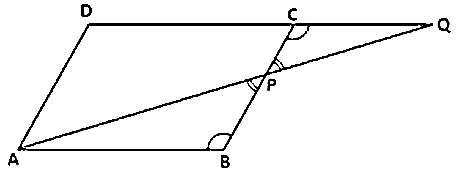We need to prove, the rectangle obtained by BP and DQ is equal to the rectangle contained by AB and BC. We need to prove that BP x DQ = AB x BC

In ΔABP and ΔQCP,

∠ABP = ΔQCP   (alternate angles as AB ∥ DC)

∠BPA = ΔQPC   (VOA)

ΔABP ∼ ΔQCP (AA similarity)

We know that corresponding sides of similar triangles are proportionalQuestion: 18

In ΔABC, AL and CM are the perpendiculars from the vertices A and C to BC and AB respect. If Al and CM intersect at O, prove that:

(i)  ΔOMA ∼ ΔOLC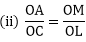Solution:(i) In ΔOMA and ΔOLC,

∠AOM = ∠COL (VOA)

∠OMA = ∠OLC (90 each)

ΔOMA ∼ ΔOLC   (A-A similarity)

(ii) Since ΔOMA ∼ ΔOLC by A-A similarity, then(Corresponding sides of similar triangles are proportional)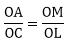Question: 19

ABCD is a quadrilateral in which AD = BC. If P, Q, R, S be the midpoints of AB, AC, CD and BD respect. Show that PQRS is a rhombus.

Solution:

Given, ABCD is a quadrilateral in which AD = BC and P, Q, R, S are the mid points of AB, AC, CD, BD, respectively.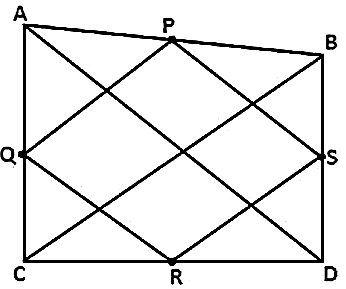To prove,

PQRS is a rhombus

Proof,

In ΔABC, P and Q are the mid points of the sides B and AC respectively

By the midpoint theorem, we get,

PQ ∥ BC, PQ = 1/2 BC.

In ΔADC, Q and R are the mid points of the sides AC and DC respectively

By the mid-point theorem, we get,

In ΔBCD,

By the mid-point theorem, we get,

RS∥BC and RS = 1/2 AD = 1/2 BC    (AD = BC)

From above eqns.

PQ = QR = RS

Thus, PQRS is a rhombus.

Question: 20

In an isosceles Δ ABC, the base AB is produced both ways to P and Q such that AP x BQ = AC2. Prove that Δ APC ∼ Δ BCQ

Solution:

Given ΔABC is isosceles and AP × BQ = AC2We need to prove that ΔAPC ∼ ΔBCQ.

Given ΔABC is an isosceles triangle AC = BC.

Now, AP × BQ = AC2 (given)

AP × BQ = AC × AC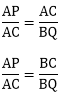Also, ∠CAB = ∠CBA   (equal sides have angles opposite to them)

180 – CAP = 180 – CBQ

∠CAP = ∠CBQ

Hence, ΔAPC ∼ ΔBCQ (SAS similarity)

Question: 21

A girl of height 90 cm is walking away from the base of a lamp post at a speed of 1.2 m/sec. If the lamp is 3.6m above the ground, find the length of her shadow after 4 seconds.

Solution:

Given, girl’s height = 90 cm, speed = 1.2m/sec and height of lamp = 3.6 mWe need to find the length of her shadow after 4 sec.

Let, AB be the lamp post and CD be the girl

Suppose DE is the length of her shadow

Let, DE = x

and BD = 1.2 × 4

BD = 4.8 m

Now, in ΔABE and ΔCDE we have,

∠B = ∠D

∠E = ∠E

So, by A-A similarity criterion,

ΔABE ∼ ΔCDE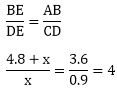3x = 4.8

x = 1.6

Hence, the length of her shadow after 4 sec. Is 1.6 m

Question: 22

A vertical stick of length 6 m casts a shadow 4m long on the ground and at the same time a tower casts a shadow 28m long. Find the height of the tower.

Solution:

Given length of vertical stick = 6 m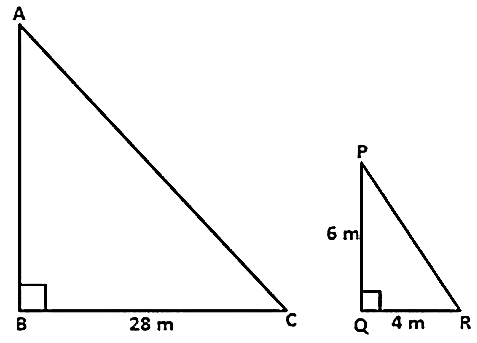We need to find the height of the tower

Suppose AB is the height of the tower and BC is its shadow.

Now, ΔABC ∼ ΔPCR (B = Q and A = P)AB = (28 × 6)/4

AB = 42 m

Hence, the height of tower is 42 m.

Question: 23

In the fig. given, ΔABC is a right angled triangle at C and DE ⊥ AB. Prove that ΔABC ∼ ΔADE.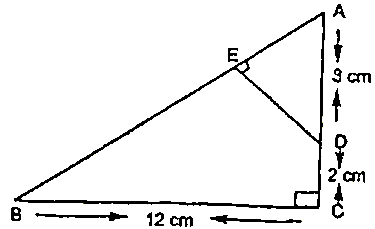Solution:

Given Δ ACB is right angled triangle and C = 90

We need to prove that ΔABC ∼ ΔADE and find the length of AE and DE.

∠A = ∠A  (common angle)

∠C = ∠E   (90)

So, by A-A similarity criterion, we have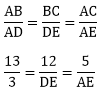Since, AB2 = AC2 + BC2

= 52 + 122

= 132

∴ DE = 36/13 cm

and   AE = 15/13 cm

Question: 24

In fig. given, we have AB ∥ CD ∥ EF. If AB = 6 cm, CD = x cm, EF = 10 cm, BD = 4 cm, and DE = y cm. Calculate the values of x and y.Solution:

Given AB CD EF.

AB = 6 cm, CD = x cm, and EF = 10 cm.

We need to calculate the values of x and y

∠ABD = ∠DEF  (alt.Interior angles)Y = 40/6

Y = 6.67 cm

Similarly, in ΔABE, we have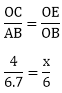6.7 × X = 6 × 4

X = 24/6.7

X = 3.75 cm

Therefore, x = 3.75 cm and y = 6.67 cm
```### Course Features

• 728 Video Lectures
• Revision Notes
• Previous Year Papers
• Mind Map
• Study Planner
• NCERT Solutions
• Discussion Forum
• Test paper with Video Solution